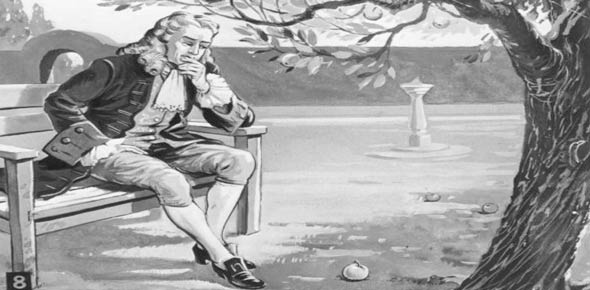# Quiz: Newton's 1st & 2nd Law

5 Questions | Attempts: 303Settings• 1.
Explain why you feel lighter when a rollercoaster pulls you downward.
• 2.
Inertia is the tendency of an object to resist changes in motion.
• A.

True

• B.

False

• 3.
What is Newton's 2nd Law of Motion?
• A.

A= (changes in Velocity)/(changes in time)

• B.

Fnet=ma, also Fw=m*g

• C.

M=f*a

• D.

A=m/f

• 4.
What do we mean when we say "net force"? -It is the _ _ _ _ _  of the forces.
• 5.
What is the acceleration of a 1.5 kg soccer ball if a 75 N net force is applied to it?
• A.

35 m/s/s

• B.

0.02 m/s/s

• C.

76.5 m/s/s

• D.

50 m/s/s

## Related TopicsBack to top
×

Wait!
Here's an interesting quiz for you.